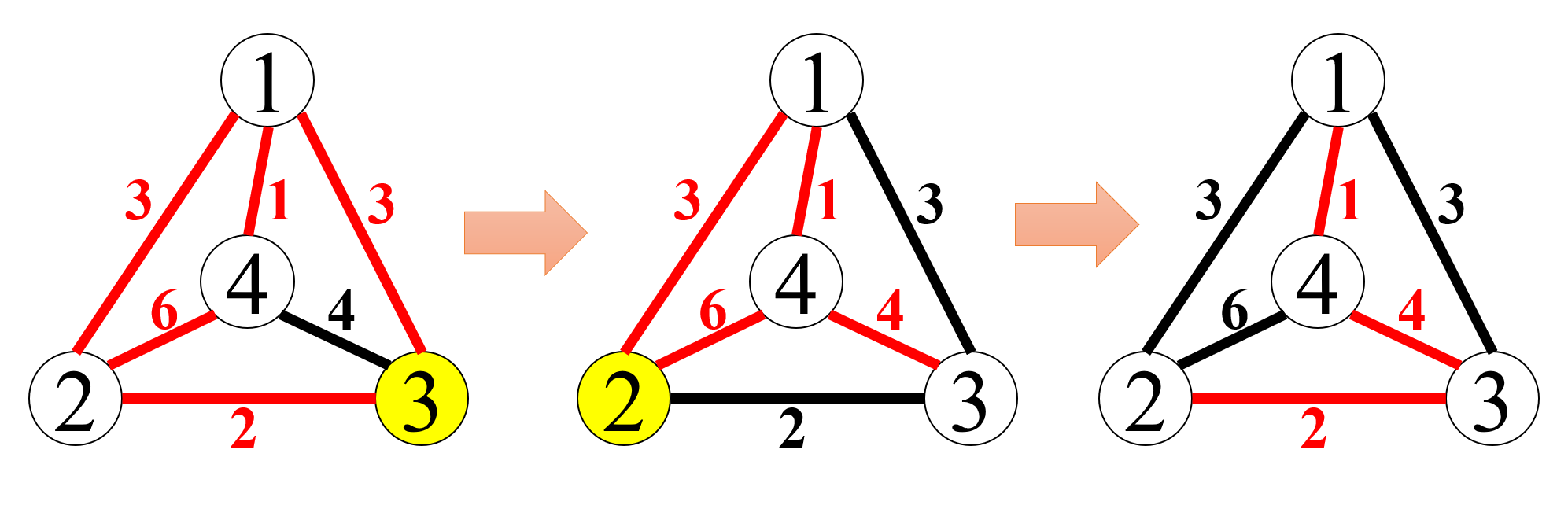시간 제한메모리 제한제출정답맞힌 사람정답 비율
2 초 512 MB1671787.547%

## 문제

You are given an undirected complete graph. Every pair of the nodes in the graph is connected by an edge, colored either red or black. Each edge is associated with an integer value called penalty.

By repeating certain operations on the given graph, a “spanning tree” should be formed with only the red edges. That is, the number of red edges should be made exactly one less than the number of nodes, and all the nodes should be made connected only via red edges, directly or indirectly. If two or more such trees can be formed, one with the least sum of penalties of red edges should be chosen.

In a single operation step, you choose one of the nodes and flip the colors of all the edges connected to it: Red ones will turn to black, and black ones to red.Fig. F-1 The first dataset of Sample Input and its solution

For example, the leftmost graph of Fig. F-1 illustrates the first dataset of Sample Input. By flipping the colors of all the edges connected to the node 3, and then flipping all the edges connected to the node 2, you can form a spanning tree made of red edges as shown in the rightmost graph of the figure.

## 입력

The input consists of multiple datasets, each in the following format.

n
e1,2 e1,3 ... e1,n-1 e1,n
e2,3 e2,4 ... e2,n
...
en-1,n


The integer n (2 ≤ n ≤ 300) is the number of nodes. The nodes are numbered from 1 to n. The integer ei,k (1 ≤ |ei,k| ≤ 105) denotes the penalty and the initial color of the edge between the node i and the node k. Its absolute value |ei,k| represents the penalty of the edge. ei,k > 0 means that the edge is initially red, and ei,k < 0 means it is black.

The end of the input is indicated by a line containing a zero. The number of datasets does not exceed 50.

## 출력

For each dataset, print the sum of edge penalties of the red spanning tree with the least sum of edge penalties obtained by the above-described operations. If a red spanning tree can never be made by such operations, print -1.

## 예제 입력 1

4
3 3 1
2 6
-4
3
1 -10
100
5
-2 -2 -2 -2
-1 -1 -1
-1 -1
1
4
-4 7 6
2 3
-1
0


## 예제 출력 1

7
11
-1
9